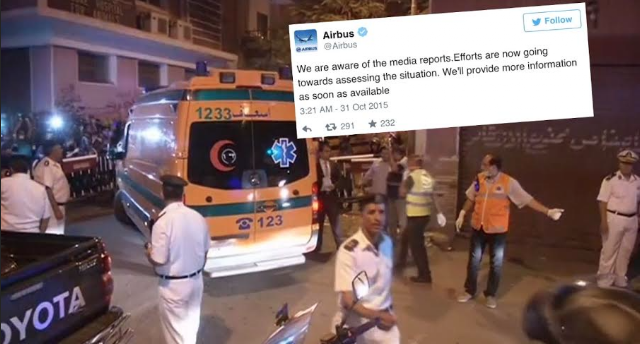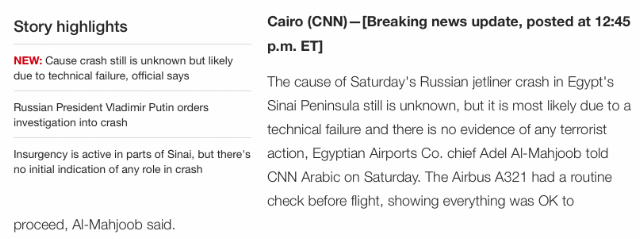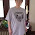## Saturday, October 31, 2015

### 25 34 73 76 106 217 224 321 | Russian Airbus A-321 Crashes, Killing 224 People On Board

It has been 224-days since March 21, or March 22, 3/22, if you count the end date.

The plane that crashed was an A-321.

Airbus A321... 321 is a lot like March 21, or 3/21.

http://www.usatoday.com/story/news/world/2015/10/31/russian-plane-crash-egypt/74934010/A part of me wonders if the 'OYOTA' without the T is intentional.Toyota = 20+15+25+15+20+1 = 96Oyota = 7610/31/2015 = 10+31+20+15 = 76
Notice the tweet time of 3:21 AM as well, about the Airbus A-321.

If you count the end date, it has been 224-days since March 22, also 3/22 or 22/3, numbers often coded into tragedy.

Further, the last reported major plane crash tragedy was in Indonesia on August 16, 2015, 76-days ago.  This is odd because today's date numerology is '76'.

10/31/2015 = 10+31+20+15 = 76Cairo = 3+1+9+18+15 = 46; Egypt = 5+7+25+16+20 = 73; Cairo, Egypt = 119
Notice the name of the man given, Adel Al-Mahjoob.  His name connects to the date of the wreck.10/31/2015 = 10+31+20+15 = 7623 minutes into the flight?
There are some interesting things about 'Sinai' and 'Red Sea'.  Notice also the mention of ISIS.

Airbus also has the Gematria of '25'.

Airbus = 1+9+9+2+3+1 = 25
Flight 9268.... 9+2+6+8 = 25

Anyhow, notice how the 217 is emphasized in the report of the children who died.

With regards to the 217 on board, check out the Gematria of these words:
Notice the Jewish Gematria of 217 and the Simple English Gematria of 76.

Today's date has a numerology of 76.

10/31/2015 = 10+31+20+15 = 76
***They say the plane was traveling 67 mph when it crashed...
***Flight MH-370 was lost on the 67th day of the year...

Notice how the word 'shocking' also has a Gematria of 217.  I think the media intends to shock audiences with these fictionalized stories, assuming this too, is another fictionalized plane crash.

Here is one more '217', that honestly, fits with most media stories.

Notice the crash location of Sharm el-Sheikh  on the Sinai Peninsula.

The population of the town of the crash connects to 'Egypt' and 'Sacrifice'.

Egypt = 5+7+25+16+20 = 73
Sacrifice = 19+1+3+18+9+6+9+3+5 = 73
(Ritual Sacrifice = 9+9+2+3+1+3+1+1+3+9+9+6+9+3+5 = 73)
The name of the company that crashed is 'MetroJet'.

MetroJet = 4+5+2+9+6+1+5+2 = 34
MetroJet = 13+5+20+18+15+10+5+20 = 106

Notice the 2:21.  It has been 221-days since the Germanwings crash of March 24, 2015, with the number 122 heavily coded, and Andreas Lubitz, the suicidal pilot, being shown in front of the Golden Gate Bridge.

And checkout the Gematria of "Andreas", for the name of the Germanwings Pilot who supposedly crashed the plane.
With regards to 62, it showed up in this incident.  At 6:20 AM, the plane disappeared off radar, 23 minutes into its flight.

Sinai Egypt = 1+9+5+1+9+5+7+7+7+2 = 53/62
St. Petersburg = 1+2+7+5+2+5+9+1+2+3+9+7 = 53/62/71
Richard Quest = 9+9+3+8+1+9+4+8+3+5+1+2 = 62/71 (CNN's Plane Story Narrator)

With regards to the Gematria of Torah, September 23 on the Chinese Lunar Calendar is upcoming November 4 on our calendar.

That span of 7 months and 7 days is also interesting.

Here are also some lose connections between; Halloween, Skull and Bones, Twenty-Five and Egypt.

1.A 321 321+123=444
Back of ambulance 123 123+321=444
Although I'm sure sure this is fake. It could be a sacrifice. Either way if it went down ISIS didn't do it. They can't hit something 31,000 feet in the air. If it was shot down my bet's on the Israelis.

1.Egypt = 73; Sacrifice = 73

2.Ritual Sacrifice =73. Israeli =73.

Sharm el-Sheik has a population of 73,000

2.IsIs Attacks Russia = America is pissed about the Syria debacle. This is a declaration of War. Hope everyone stays safe at That Mets vs. Royals game tonight. Game 1 blackout was beyond strange.

3.Def did your homework on this one Zach!